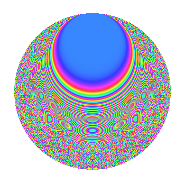Properties

 Label 1175.4.aLevel 1175 Weight 4 Character orbit a Rep. character $$\chi_{1175}(1,\cdot)$$ Character field $$\Q$$ Dimension 219 Newforms 12 Sturm bound 480 Trace bound 2

Related objects

Defining parameters

 Level: $$N$$ = $$1175 = 5^{2} \cdot 47$$ Weight: $$k$$ = $$4$$ Character orbit: $$[\chi]$$ = 1175.a (trivial) Character field: $$\Q$$ Newforms: $$12$$ Sturm bound: $$480$$ Trace bound: $$2$$ Distinguishing $$T_p$$: $$2$$

Dimensions

The following table gives the dimensions of various subspaces of $$M_{4}(\Gamma_0(1175))$$.

Total New Old
Modular forms 366 219 147
Cusp forms 354 219 135
Eisenstein series 12 0 12

The following table gives the dimensions of the cuspidal new subspaces with specified eigenvalues for the Atkin-Lehner operators and the Fricke involution.

$$5$$$$47$$FrickeDim.
$$+$$$$+$$$$+$$$$59$$
$$+$$$$-$$$$-$$$$44$$
$$-$$$$+$$$$-$$$$53$$
$$-$$$$-$$$$+$$$$63$$
Plus space$$+$$$$122$$
Minus space$$-$$$$97$$

Trace form

 $$219q$$ $$\mathstrut -\mathstrut 6q^{2}$$ $$\mathstrut +\mathstrut 2q^{3}$$ $$\mathstrut +\mathstrut 890q^{4}$$ $$\mathstrut +\mathstrut 32q^{6}$$ $$\mathstrut +\mathstrut 18q^{7}$$ $$\mathstrut -\mathstrut 30q^{8}$$ $$\mathstrut +\mathstrut 1959q^{9}$$ $$\mathstrut +\mathstrut O(q^{10})$$ $$219q$$ $$\mathstrut -\mathstrut 6q^{2}$$ $$\mathstrut +\mathstrut 2q^{3}$$ $$\mathstrut +\mathstrut 890q^{4}$$ $$\mathstrut +\mathstrut 32q^{6}$$ $$\mathstrut +\mathstrut 18q^{7}$$ $$\mathstrut -\mathstrut 30q^{8}$$ $$\mathstrut +\mathstrut 1959q^{9}$$ $$\mathstrut +\mathstrut 70q^{11}$$ $$\mathstrut -\mathstrut 7q^{12}$$ $$\mathstrut -\mathstrut 40q^{13}$$ $$\mathstrut +\mathstrut 205q^{14}$$ $$\mathstrut +\mathstrut 3538q^{16}$$ $$\mathstrut +\mathstrut 138q^{17}$$ $$\mathstrut +\mathstrut 35q^{18}$$ $$\mathstrut -\mathstrut 70q^{19}$$ $$\mathstrut -\mathstrut 64q^{21}$$ $$\mathstrut +\mathstrut 218q^{22}$$ $$\mathstrut -\mathstrut 24q^{23}$$ $$\mathstrut +\mathstrut 863q^{24}$$ $$\mathstrut -\mathstrut 476q^{26}$$ $$\mathstrut +\mathstrut 56q^{27}$$ $$\mathstrut +\mathstrut 660q^{28}$$ $$\mathstrut -\mathstrut 496q^{29}$$ $$\mathstrut -\mathstrut 132q^{31}$$ $$\mathstrut +\mathstrut 339q^{32}$$ $$\mathstrut +\mathstrut 432q^{33}$$ $$\mathstrut -\mathstrut 216q^{34}$$ $$\mathstrut +\mathstrut 7618q^{36}$$ $$\mathstrut +\mathstrut 566q^{37}$$ $$\mathstrut -\mathstrut 820q^{38}$$ $$\mathstrut +\mathstrut 460q^{39}$$ $$\mathstrut +\mathstrut 226q^{41}$$ $$\mathstrut +\mathstrut 463q^{42}$$ $$\mathstrut +\mathstrut 402q^{43}$$ $$\mathstrut -\mathstrut 1316q^{44}$$ $$\mathstrut -\mathstrut 874q^{46}$$ $$\mathstrut -\mathstrut 235q^{47}$$ $$\mathstrut -\mathstrut 860q^{48}$$ $$\mathstrut +\mathstrut 10855q^{49}$$ $$\mathstrut -\mathstrut 222q^{51}$$ $$\mathstrut -\mathstrut 60q^{52}$$ $$\mathstrut +\mathstrut 454q^{53}$$ $$\mathstrut +\mathstrut 1125q^{54}$$ $$\mathstrut +\mathstrut 3244q^{56}$$ $$\mathstrut +\mathstrut 764q^{57}$$ $$\mathstrut +\mathstrut 520q^{58}$$ $$\mathstrut +\mathstrut 162q^{59}$$ $$\mathstrut +\mathstrut 2542q^{61}$$ $$\mathstrut +\mathstrut 324q^{62}$$ $$\mathstrut +\mathstrut 1628q^{63}$$ $$\mathstrut +\mathstrut 14134q^{64}$$ $$\mathstrut +\mathstrut 2068q^{66}$$ $$\mathstrut +\mathstrut 658q^{67}$$ $$\mathstrut +\mathstrut 2113q^{68}$$ $$\mathstrut -\mathstrut 1532q^{69}$$ $$\mathstrut +\mathstrut 522q^{71}$$ $$\mathstrut -\mathstrut 1254q^{72}$$ $$\mathstrut -\mathstrut 2462q^{73}$$ $$\mathstrut +\mathstrut 3064q^{74}$$ $$\mathstrut -\mathstrut 3750q^{76}$$ $$\mathstrut +\mathstrut 1556q^{77}$$ $$\mathstrut -\mathstrut 824q^{78}$$ $$\mathstrut -\mathstrut 1498q^{79}$$ $$\mathstrut +\mathstrut 20595q^{81}$$ $$\mathstrut +\mathstrut 2126q^{82}$$ $$\mathstrut +\mathstrut 1440q^{83}$$ $$\mathstrut -\mathstrut 1199q^{84}$$ $$\mathstrut +\mathstrut 2036q^{86}$$ $$\mathstrut -\mathstrut 4408q^{87}$$ $$\mathstrut +\mathstrut 2920q^{88}$$ $$\mathstrut +\mathstrut 3398q^{89}$$ $$\mathstrut +\mathstrut 1508q^{91}$$ $$\mathstrut +\mathstrut 84q^{92}$$ $$\mathstrut +\mathstrut 6484q^{93}$$ $$\mathstrut +\mathstrut 376q^{94}$$ $$\mathstrut -\mathstrut 1484q^{96}$$ $$\mathstrut +\mathstrut 2738q^{97}$$ $$\mathstrut -\mathstrut 2234q^{98}$$ $$\mathstrut +\mathstrut 5542q^{99}$$ $$\mathstrut +\mathstrut O(q^{100})$$

Decomposition of $$S_{4}^{\mathrm{new}}(\Gamma_0(1175))$$ into irreducible Hecke orbits

Label Dim. $$A$$ Field CM Traces A-L signs $q$-expansion
$$a_2$$ $$a_3$$ $$a_5$$ $$a_7$$ 5 47
1175.4.a.a $$3$$ $$69.327$$ 3.3.1101.1 None $$5$$ $$5$$ $$0$$ $$45$$ $$+$$ $$+$$ $$q+(2+\beta _{2})q^{2}+(1+\beta _{1}-\beta _{2})q^{3}+(2+\cdots)q^{4}+\cdots$$
1175.4.a.b $$8$$ $$69.327$$ $$\mathbb{Q}[x]/(x^{8} - \cdots)$$ None $$-3$$ $$-7$$ $$0$$ $$-39$$ $$+$$ $$-$$ $$q-\beta _{1}q^{2}+(-1-\beta _{5})q^{3}+(5+\beta _{2}-\beta _{3}+\cdots)q^{4}+\cdots$$
1175.4.a.c $$8$$ $$69.327$$ $$\mathbb{Q}[x]/(x^{8} - \cdots)$$ None $$3$$ $$10$$ $$0$$ $$15$$ $$+$$ $$-$$ $$q+\beta _{1}q^{2}+(2-\beta _{1}-\beta _{3})q^{3}+(1+\beta _{1}+\cdots)q^{4}+\cdots$$
1175.4.a.d $$10$$ $$69.327$$ $$\mathbb{Q}[x]/(x^{10} - \cdots)$$ None $$-7$$ $$-8$$ $$0$$ $$-9$$ $$+$$ $$-$$ $$q+(-1+\beta _{1})q^{2}+(-1+\beta _{6})q^{3}+(4+\cdots)q^{4}+\cdots$$
1175.4.a.e $$13$$ $$69.327$$ $$\mathbb{Q}[x]/(x^{13} - \cdots)$$ None $$3$$ $$10$$ $$0$$ $$19$$ $$+$$ $$+$$ $$q+\beta _{1}q^{2}+(1+\beta _{4})q^{3}+(4+\beta _{2})q^{4}+\cdots$$
1175.4.a.f $$15$$ $$69.327$$ $$\mathbb{Q}[x]/(x^{15} - \cdots)$$ None $$-7$$ $$-8$$ $$0$$ $$-13$$ $$+$$ $$+$$ $$q-\beta _{1}q^{2}+(-1-\beta _{4})q^{3}+(5+\beta _{2})q^{4}+\cdots$$
1175.4.a.g $$18$$ $$69.327$$ $$\mathbb{Q}[x]/(x^{18} - \cdots)$$ None $$-1$$ $$-7$$ $$0$$ $$-6$$ $$+$$ $$-$$ $$q-\beta _{1}q^{2}-\beta _{4}q^{3}+(3+\beta _{2})q^{4}+(-1+\cdots)q^{6}+\cdots$$
1175.4.a.h $$18$$ $$69.327$$ $$\mathbb{Q}[x]/(x^{18} - \cdots)$$ None $$1$$ $$7$$ $$0$$ $$6$$ $$-$$ $$+$$ $$q+\beta _{1}q^{2}+\beta _{4}q^{3}+(3+\beta _{2})q^{4}+(-1+\cdots)q^{6}+\cdots$$
1175.4.a.i $$28$$ $$69.327$$ None $$-1$$ $$-7$$ $$0$$ $$-6$$ $$+$$ $$+$$
1175.4.a.j $$28$$ $$69.327$$ None $$1$$ $$7$$ $$0$$ $$6$$ $$-$$ $$-$$
1175.4.a.k $$35$$ $$69.327$$ None $$-8$$ $$-12$$ $$0$$ $$-84$$ $$-$$ $$+$$
1175.4.a.l $$35$$ $$69.327$$ None $$8$$ $$12$$ $$0$$ $$84$$ $$-$$ $$-$$

Decomposition of $$S_{4}^{\mathrm{old}}(\Gamma_0(1175))$$ into lower level spaces

$$S_{4}^{\mathrm{old}}(\Gamma_0(1175)) \cong$$ $$S_{4}^{\mathrm{new}}(\Gamma_0(5))$$$$^{\oplus 4}$$$$\oplus$$$$S_{4}^{\mathrm{new}}(\Gamma_0(25))$$$$^{\oplus 2}$$$$\oplus$$$$S_{4}^{\mathrm{new}}(\Gamma_0(47))$$$$^{\oplus 3}$$$$\oplus$$$$S_{4}^{\mathrm{new}}(\Gamma_0(235))$$$$^{\oplus 2}$$